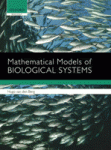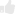# Book review: An introduction to mathematical biology

Posted by , on 15 December 2011

This book review originally appeared in Development. Lance Davidson reviews “Mathematical Models of Biological Systems” (by Hugo van den Berg).

Book info:
Mathematical Models of Biological Systems By Hugo van den Berg Oxford University Press (2010) 256 pages ISBN 978-0-19-958218-1 (paperback), 978-0-19-958219-8 (hardback) £27.50/\$49.50 (paperback), £65/\$117 (hardback)One of the key goals of modern cell and developmental biology is to expose the underlying principles that drive cell differentiation and to elucidate how organisms construct functional multicellular structures. Thanks to advances in sequencing, high throughput screens and sophisticated imaging technologies, these fields are now awash with quantitative descriptions of gene transcription, cell signaling and cell mechanics. However, extracting key principles from the flood of new data is a major challenge for researchers and a central obstacle to fundamental progress in cell and developmental biology. The tools required to interpret this vast amount of biological data and to test hypotheses based on these studies can be found in quantitative analysis and mathematical modeling. With the book Mathematical Models of Biological Systems, Hugo van den Berg aims to contribute to the training of a new generation of biologists and mathematicians and to provide them with an introduction to the methods that are now available to quantitatively analyze biological data.

Like many quantitative biologists, my first exposure to mathematical modeling was not in the context of cell biology or developmental biology, but came through examples from physical chemistry, physiology and population ecology. In these fields, simple problems can be formulated using ordinary differential equations (ODEs) with complete statements of the state variables, such as initial conditions. As students, we learned to write ‘word-models’ and to translate these into sets of ODEs. Word models are narrative passages intended to translate the details of a biological problem such that biologists and mathematicians alike can understand the problem in a way that allows equations to be written which capture those details. For instance, we can distil the interactions between predators and prey by stating the rules that govern their populations. Rules that govern the population of prey might include sources of population growth, such as birth or migration, and losses to the population due to predation or disease. The precise statement of these rules should be complete enough to govern the mathematical formulation of the model. Given a well-defined word model, the mathematical biologist can then write a series of ODEs; for example, with variables that represent the number of predators and prey and equations to describe how populations of predators and prey change. As students, we sometimes discovered that there were closed form solutions of these ODEs, in which changes in variables can be predicted explicitly by equations. But more often we found that we could only evaluate the general dynamic behavior of the variables; for instance, whether populations of predators and prey are stable or not. The insights and training that these model-building exercises gave us were instrumental in becoming fluent in the basic skills of mathematical modeling. The processes of formulating a model and relating fundamental principles to the mathematics and experimental outcomes were often more informative than the solution itself. However, after marveling at the awesome power of ODEs, we soon realized that the solution of some, or indeed most sets of, ODEs was intractable, that there was no way to capture relevant details of complex biology with continuous variables, or that model predictions could not be tested experimentally. As such, the tool kit of ODEs used to learn the skills of mathematical modeling is less useful for developing the quantitative models that are needed to describe problems in cell and developmental biology.

Unlike physics or chemistry, biology has very few universal theories. In classical physics, theoretical frameworks can connect multiple experimental fields. By contrast, most models in mathematical biology are developed ad hoc to describe a single series of experiments. To think that a slim textbook could capture the entirety of mathematical biology, with all its ad hoc models, would be absurd, but this book provides a good introduction to it by presenting classical applications of ODEs. The first half of the book introduces the reader to the mathematical tool kit needed to formulate models and to solve or characterize their behavior. Most of the second half focuses on specific applications of modeling, with chapters on population growth (ecology), dynamics of disease in populations (epidemiology) and within the individual (immunology), and physiology. Many of the examples are similar to those found in classical texts, such as James Murray’s series on Mathematical Biology (Murray, 2007), Leah Edelstein-Keshet’s Mathematical Models in Biology (Edelstein-Keshet, 2005) or Lee Segel’s excellent Modeling Dynamic Phenomena in Molecular and Cellular Biology (Segel, 1984). Despite some organizational flaws, such as the placement of a chapter on the philosophy of mathematical modeling in the middle of the book, and the introduction of stochastic processes at the end of the book, developmental biologists may be interested in using van den Berg’s book to explore the basic principles of mathematical modeling; for example, for developing word models and methods for solving and analyzing systems of ODEs. From the perspective of a developmental biologist, a glaring omission from this book is any discussion of pattern formation or examples of diffusion-reaction equations. Early work on partial differential equations and pattern formation by Alan Turing in the 1950s (Turing, 1952) and Hans Meinhardt in the 1970s (Gierer and Meinhardt, 1972; Meinhardt, 1977) provided the stimulus for many theorists to enter the field of developmental biology and attracted quantitative biologists to challenge those models. Given the lack of textbooks focused on mathematical models in developmental biology, students interested in modeling the complex problems of modern cell and developmental biology would be well advised to augment this text with other books on modeling specific biophysical systems and with more targeted reading of extensive supplemental materials that accompany the primary literature of gene regulatory networks, cell signaling and morphogenesis.

Current state-of-the-art modeling efforts in developmental biology range from Bayesian models to finite element models to agent-based models, but the model-building exercises and focus on ODEs presented in van den Berg’s text are still essential to form an understanding of the field of mathematical modeling in biology. The tutorial forms presented by van den Berg provide a concise introduction to the principles of modeling and dynamical systems and are suitable for an introductory course in mathematical biology. However, more advanced students and quantitatively inclined developmental biologists will have to wait for textbooks that provide a more complete introduction to computational tools that have seen extensive application in recent years.(1 votes)Loading...

Tags: ,
Categories: Book Reviews